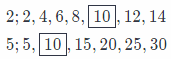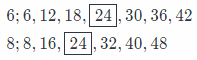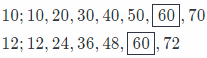# Texas Go Math Grade 6 Module 2 Answer Key Rational Numbers

Refer to our Texas Go Math Grade 6 Answer Key Pdf to score good marks in the exams. Test yourself by practicing the problems from Texas Go Math Grade 6 Module 2 Answer Key Rational Numbers.

## Texas Go Math Grade 6 Module 2 Answer Key Rational Numbers

Write each improper fraction as a mixed number.

Question 1.
$$\frac{7}{2}$$
Firstly, we will write this improper fraction as a sum of proper fractions(ones) plus one which is not proper
SecondLy, we wilt exchange proper fractions with ones!
Final soLution wilt be: mixed number.

$$\frac{7}{2}$$ = $$\frac{2}{2}+\frac{2}{2}+\frac{2}{2}+\frac{1}{2}$$
= 1 + 1 + 1 + $$\frac{1}{2}$$
= 3 + $$\frac{1}{2}$$
= 3$$\frac{1}{2}$$

Question 2.
$$\frac{12}{5}$$
Firstly, we will write this improper fraction as a sum of proper fractions(ones) plus one which is not proper
Secondly, we will exchange proper fractions with ones!
Final solution will be: mixed number

$$\frac{12}{5}$$ = $$\frac{5}{5}+\frac{5}{5}+\frac{2}{5}$$
= 1 + 1 + $$\frac{2}{5}$$
= 2 + $$\frac{2}{5}$$
= 2$$\frac{2}{5}$$

Question 3.
$$\frac{11}{7}$$
Firstly, we will write this improper fraction as a sum of proper fractions(ones) plus one which is not proper
SecondLy, we wiLl exchange proper fractions with ones!
Final solution will be: mixed number

$$\frac{11}{7}$$ = $$\frac{7}{7}+\frac{4}{7}$$
= 1 + $$\frac{4}{7}$$
= 1$$\frac{4}{7}$$

Question 4.
$$\frac{15}{4}$$
Firstly, we will write this improper fraction as a sum of proper fractions(ones) plus one which is not proper
Secondly, we will exchange proper fractions with ones!
Final solution will be: mixed number.

$$\frac{15}{4}$$ = $$\frac{4}{4}+\frac{4}{4}+\frac{4}{4}+\frac{3}{4}$$
= 1 + 1 + 1 + $$\frac{3}{4}$$
= 3 + $$\frac{3}{4}$$
= 3$$\frac{3}{4}$$

Write each mixed number as an improper fraction.

Question 5.
2$$\frac{1}{2}$$
Firstly, we will write the whole number as a sum of ones.
Secondly, we will use the denominator to find corresponding fractions for ones.
Thirdly, we will. calculate sum of numerators!
To reach our final solution: improper fraction.

2$$\frac{1}{2}$$ = 1 + 1 + $$\frac{1}{2}$$
= $$\frac{2}{2}+\frac{2}{2}+\frac{1}{2}$$
= $$\frac{5}{2}$$

Question 6.
4$$\frac{3}{5}$$
Firstly, we will write the whole number as a sum of ones.
Secondly, we will use the denominator to find corresponding fractions for ones.
Thirdly, we will calculate sum of numerators!
To reach our final solution: improper fraction.

4$$\frac{3}{5}$$ = 1 + 1 + 1 + 1 + $$\frac{3}{5}$$
= $$\frac{5}{5}+\frac{5}{5}+\frac{5}{5}+\frac{5}{5}+\frac{3}{5}$$
= $$\frac{23}{5}$$

Question 7.
3$$\frac{4}{9}$$
Firstly, we will write the whole number as a sum of ones.
Secondly, we will use the denominator to find corresponding fractions for ones.
Thirdly, we will calculate sum of numerators!
To reach our final solution: improper fraction.

3$$\frac{4}{9}$$ = 1 + 1 + 1 + $$\frac{4}{9}$$
= $$\frac{9}{9}+\frac{9}{9}+\frac{9}{9}+\frac{4}{9}$$
= $$\frac{31}{9}$$

Question 8.
2$$\frac{5}{7}$$
Firstly, we will write the whole number as a sum of ones.
Secondly, we will use the denominator to find corresponding fractions for ones.
Thirdly, we will calculate sum of numerators!
To reach our final solution: improper fraction.

2$$\frac{5}{7}$$ = 1 + 1 + $$\frac{5}{7}$$
= $$\frac{7}{7}+\frac{7}{7}+\frac{5}{7}$$
= $$\frac{19}{7}$$

Find the least common denominator.

Question 9.
$$\frac{1}{2}$$ and $$\frac{3}{5}$$ _________
Firstly, we will list multiples of each denominator.
Secondly, we will highlight common multiples!
Thirdly, we will determine, which is the least common denominator!Least Common denominator is 10

Question 10.
$$\frac{1}{6}$$ and $$\frac{3}{8}$$ _________
Firstly, we will list multiples of each denominator.
Secondly, we will highlight common multiples!
Thirdly, we will determine, which is the least common denominator!Least Common denominator is 24

Question 11.
$$\frac{9}{10}$$ and $$\frac{7}{12}$$ _________
Firstly, we will list multiples of each denominator.
Secondly, we will highlight common multiples!
Thirdly, we will determine, which is the least common denominator!Least Common denominator is 60

Question 12.
$$\frac{4}{9}$$ and $$\frac{5}{12}$$ _________
Firstly, we will list multiples of each denominator.
Secondly, we will highlight common multiples!
Thirdly, we will determine, which is the least common denominator!Least Common denominator is 36

Visualize Vocabulary
Use the ✓ words to complete the web. You may put more than one word in each box.Understand Vocabulary

Fill in each blank with the correct term from the preview words.

Question 1.
A _________________ is any number that can be written as a ratio of two integers.